# CD Ackermann VI

LabVIEW 2018 Control Design and Simulation Module Help

Edition Date: March 2018

Part Number: 371894J-01

»View Product InfoDownload Help (Windows Only)

Owning Palette: State Feedback Design VIs

Requires: Control Design and Simulation Module

Uses the Ackermann formula with the controllability matrix to determine the controller feedback gain matrix K that places the closed-loop poles in the locations you specify. You also can use this VI to determine the observer gain matrix L that places the observer poles in the locations you specify. You can use the CD Ackermann VI with single-input multiple-output (SIMO) or multiple-input single-output (MISO) systems when placing closed-loop control or observer poles, respectively.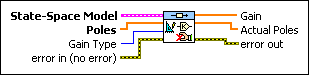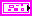State-Space Model contains a mathematical representation of and information about the system of which this VI places closed-loop poles in desired locations using the Gain in the state feedback. You also can use pole placement to calculate the estimator gain and set the pole locations of the full state observer.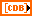Poles specifies the closed-loop pole locations.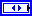Gain Type specifies the type of gain this VI returns in the Gain parameter. Use the Controller Gain with the CD State-Space Controller VI. Use the Observer Gain (Predictive) and Observer Gain (Current) with the CD Discrete Observer function and the Continuous Observer function.

For discrete systems, the Observer Gain (Predictive) relates to the Observer Gain (Current) through the following relationship:

Observer Gain (Predictive) = A . Observer Gain (Current).

For continuous systems, the notion of current and predictive time does not apply, so specifying either observer gain returns the correct gain value.

 0 Controller Gain (default)—Calculates feedback gain K for a controller, such that the controller pole locations are the locations you specify using the Poles parameter. The system must be a single-input system and controllable, meaning the controllability matrix must be non-singular. 1 Observer Gain (Predictive)—Calculates feedback gain Lp for a predictive observer, such that the observer pole locations are the locations you specify using the Poles parameter. The system must be a single-output system and observable, meaning the observability matrix must be non-singular. 2 Observer Gain (Current)—Calculates feedback gain Lc for a current observer, such that the observer pole locations are the locations you specify using the Poles parameter. The system must be a single-output system and observable, meaning the observability matrix must be non-singular. For continuous systems, the notion of current and predictive time does not apply; either observer gain returns the correct gain value. Also, if the system is discrete, A must be invertible.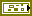error in describes error conditions that occur before this node runs. This input provides standard error in functionality.Gain returns the feedback gain, according to the value you specify for the Gain Type parameter, that produces a closed-loop system such that the poles are equal to the values you specify in the Poles parameter.Actual Poles are the eigenvalues of the closed-loop system matrix Ã. The definition of Ã depends on the value you specify for the Gain Type parameter.

If Gain Type is Controller Gain, Ã = ABK.

If Gain Type is Observer Gain (Predictive), Ã = ALC, where L is the Gain this VI returns.

If Gain Type is Observer Gain (Current), Ã = ALCA.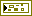error out contains error information. This output provides standard error out functionality.

## CD Ackermann Details

This VI does not support delays unless the delays are part of the mathematical model that represents the dynamic system. To account for the delays in the synthesis of the controller or state estimator, you must incorporate the delays into the mathematical model of the dynamic system using the CD Convert Delay with Pade Approximation VI (continuous models) or the CD Convert Delay to Poles at Origin VI (discrete models). Refer to the LabVIEW Control Design User Manual for more information about delays and the limitations of Pade Approximation.